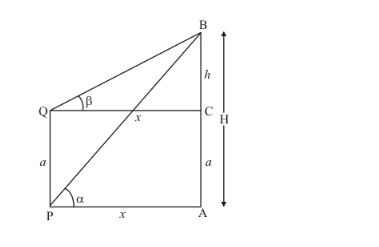# PQ is a post of given height a, and AB is a tower at some distance.

Question:

PQ is a post of given height a, and AB is a tower at some distance. If α and β are the angles of elevation of B, the top of the tower, at P and Q respectively. Find the height of the tower and its distance from the post.

Solution:

Let AB be the tower of height H and PQ is a given post of height a ,andare angles of elevation of top of towerfrom P and Q. Let PA = x.and BC = h.

The corresponding figure is as followsIn ∆QCB,

$\Rightarrow \quad \tan \beta=\frac{h}{x}$

$\Rightarrow \quad x=\frac{h}{\tan \beta}$

Again in ∆PAB,

$\Rightarrow \quad \tan \alpha=\frac{h+a}{x}$

$\Rightarrow \quad \tan \alpha=\frac{(h+a) \tan \beta}{h}$

$\Rightarrow \quad h \tan \alpha=(h+a) \tan \beta$

$\Rightarrow h(\tan \alpha-\tan \beta)=a \tan \beta$

$\Rightarrow \quad h=\frac{a \tan \beta}{\tan \alpha-\tan \beta}$

Now

$\Rightarrow \quad x=\frac{a \tan \beta}{(\tan \alpha-\tan \beta) \times \tan \beta}$

$\Rightarrow \quad x=\frac{a}{\tan \alpha-\tan \beta}$

$\Rightarrow \quad H=a+\frac{a \tan \beta}{\tan \alpha-\tan \beta}$

$\Rightarrow \quad H=\frac{a \tan \alpha}{\tan \alpha-\tan \beta}$

Hence required height is $\frac{a \tan \alpha}{\tan \alpha-\tan \beta}$. And distance is $\frac{a}{\tan \alpha-\tan \beta}$